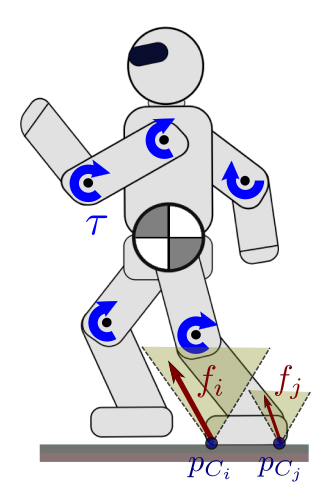# Constrained equations of motion

This page details how the Jacobian-transpose terms that map external forces to joint torques appear in the equations of motion of legged robots in contact with their environment.

## DerivationWe consider a legged robot, for instance a biped, making point or surface contacts with its environment. These kinematic constraints can be summarized into a holonomic vector equality constraint $$\bff(\bfq) = \bfzero$$. For instance, the function $$\bff(\bfq)$$ might stack contact point equality constraints of the form $$\bfp_C(\bfq) = \bfp_{D}$$ with $$\bfp_D$$ fixed in the inertial frame. Differentiating $$\bff(\bfq) = \bfzero$$ twice with respect to time yields:

\begin{equation*} \bfJ(\bfq) \qdd + \qd^\top \bfH(\bfq) \qd = \boldsymbol{0} \end{equation*}

with $$\bfJ = ({\partial \bff} / {\partial \bfq})$$ is the Jacobian matrix of the contact constraint and $$\bfH = ({\partial^2 \bff} / {\partial \bfq^2})$$ is its Hessian.

### Equations of free motion

In the absence of constraint, the joint-space equations of motion of our robot would be:

\begin{equation*} \bfM(\bfq) \qdd_{\mathrm{free}} + \qd^\top \bfC(\bfq) \qd = \bfS^\top \bftau + \bftau_g(\bfq) + \bftau_{\mathit{ext}} \end{equation*}

where:

• $$\bfM(\q)$$ is the $$n \times n$$ joint-space inertia matrix,
• $$\bfC(\q)$$ is the $$n \times n \times n$$ joint-space Coriolis tensor,
• $$\bftau$$ is the $$n$$-dimensional vector of actuated joint torques,
• $$\bftau_g(\q)$$ is the $$n$$-dimensional vector of gravity torques,
• $$\bftau_{\mathit{ext}}$$ is an optional $$n$$-dimensional vector of joint torques caused by other external forces.

We know how to compute these matrices and vectors from link inertias and Jacobians.

### Principle of least constraint

When the robot is in contact, its motion doesn't follow the vector $$\qdd_{\mathrm{free}}$$ defined by this equation because of the additional contact constraint: the actual $$\qdd$$ has to be such that

\begin{equation*} \bfJ(\bfq) \qdd + \qd^\top \bfH(\bfq) \qd = \boldsymbol{0} \end{equation*}

Gauss's principle of least constraint states that the robot's actual acceleration $$\qdd$$ is as close as possible to the free acceleration $$\qdd_{\mathrm{free}}$$, subject to this constraint:

\begin{equation*} \begin{array}{rl} \qdd = \arg\min_{\bfa} & \frac{1}{2} \| \bfa - \qdd_{\mathrm{free}}\|_{\bfM}^2 \\ \textrm{subject to} & \bfJ(\bfq) \bfa + \qd^\top \bfH(\bfq) \qd = \boldsymbol{0} \end{array} \end{equation*}

Note how the inertia matrix appears in the objective function to define what as close as possible means here. (The inertia matrix is positive definite so this objective function is strictly convex.) We can write the Lagrangian of this equality-constrained least-squares problem as:

\begin{equation*} \calL(\bfa, \bflambda) = \frac{1}{2} (\bfa - \qdd_{\mathrm{free}})^\top \bfM (\bfa - \qdd_{\mathrm{free}}) - \bflambda^\top \left(\bfJ \bfa + \qd^\top \bfH \qd\right) \end{equation*}

where we omit dependencies on $$\bfq$$ for concision. Dual variables stacked in the vector $$\bflambda$$ correspond to contact forces. Why? By definition! In Lagrangian mechanics, there are two kinds of forces: constraint and non-constraint forces. Non-constraint forces are somehow easier to deal with: they correspond to the vectors $$\bftau_g(\bfq)$$ and $$\bftau_{\mathit{ext}}$$ in the equations of free motion, and we already know how to compute them. Contact forces are the constraint forces arising from our kinematic contact constraint.

We can think of forces as dual variables that appear in the equations of motion to ensure that the system's motion ends up satisfying the kinematic constraint. Our assumption here is that the constraint will hold, i.e. that the robot will stay in contact with its environment. In practice this is not true for any command: if we send maximum joint torques, our robot will likely shake and jump all over the place, breaking the assumption of fixed contact. To make sure that this assumption holds, we will complement our kinematic constraint with a dynamic contact stability condition, but this is another story. Let us get back to our derivation.

### Method of Lagrange multipliers

From the method of Lagrange multipliers, the optimum $$(\bfa^*, \bflambda^*)$$ of our constrained problem is a critical point of the Lagrange function:

\begin{equation*} \begin{array}{ccc} \displaystyle \frac{\partial \calL}{\partial \bfa}(\bfa^*, \bflambda^*) = \bfzero & \wedge & \displaystyle \frac{\partial \calL}{\partial \bflambda}(\bfa^*, \bflambda^*) = \bfzero \end{array} \end{equation*}

The second equation is, by construction, our contact constraint. The first one gives us:

\begin{equation*} \bfM (\bfa^* - \qdd_{\mathrm{free}}) - \bfJ^T \bflambda^* = \bfzero \end{equation*}

In fine, replacing $$\bfa^*$$ by our notation for joint accelerations $$\qdd$$ and similarly $$\bflambda^*$$ by $$\bff$$, we obtain the constrained equations of motion in joint space:

\begin{equation*} \bfM(\bfq) \qdd + \qd^\top \bfC(\bfq) \qd = \bfS^\top \bftau + \bftau_g(\bfq) + \bftau_\mathit{ext} + \bfJ^\top \bff \end{equation*}

with $$\bfM(\q)$$ the joint-space inertia matrix, $$\bfC(\q)$$ the joint-space Coriolis tensor, $$\bftau_g(\q)$$ the vector of gravity torques, $$\bftau$$ the vector of actuated joint torques, $$\bfJ$$ the stacked Jacobian of kinematic contact constraints and $$\bff$$ the corresponding stacked vector of contact forces.

## To go further

This page is based on Wieber's Some comments on the structure of the dynamics of articulated motion, as well as on very good a summary in Section IV.A of this paper by Budhiraja et al.

Pages of this website are under the CC-BY 4.0 license.